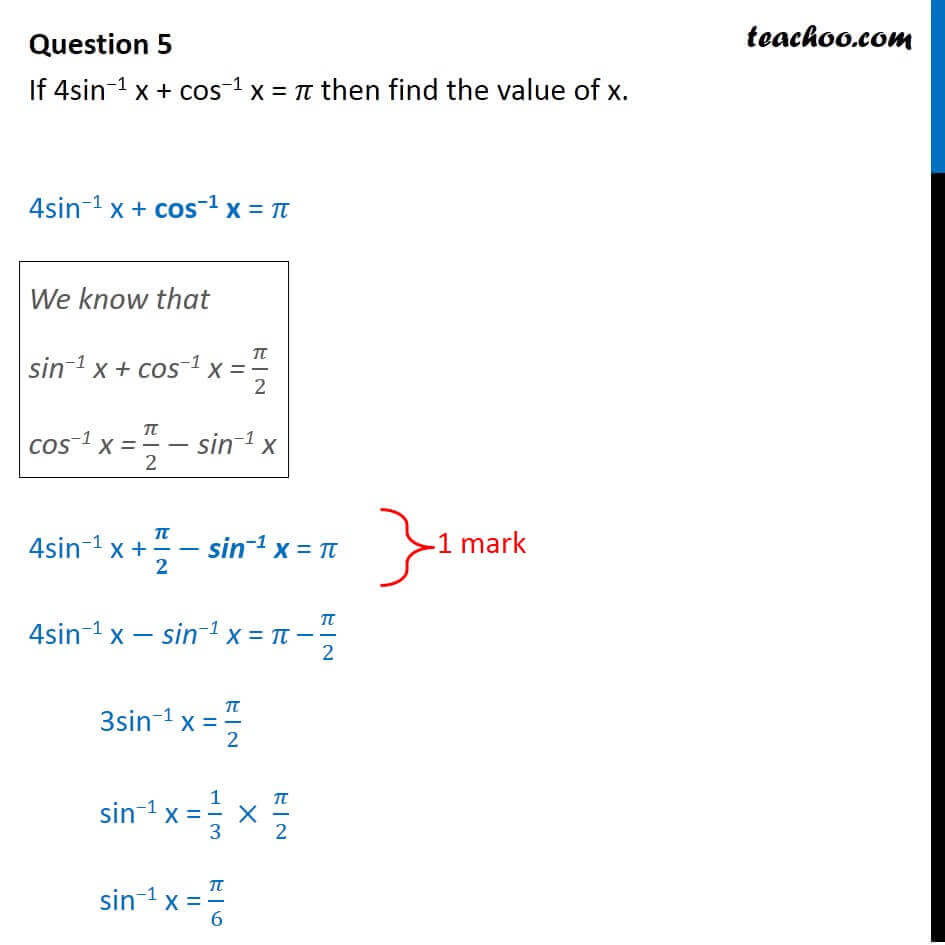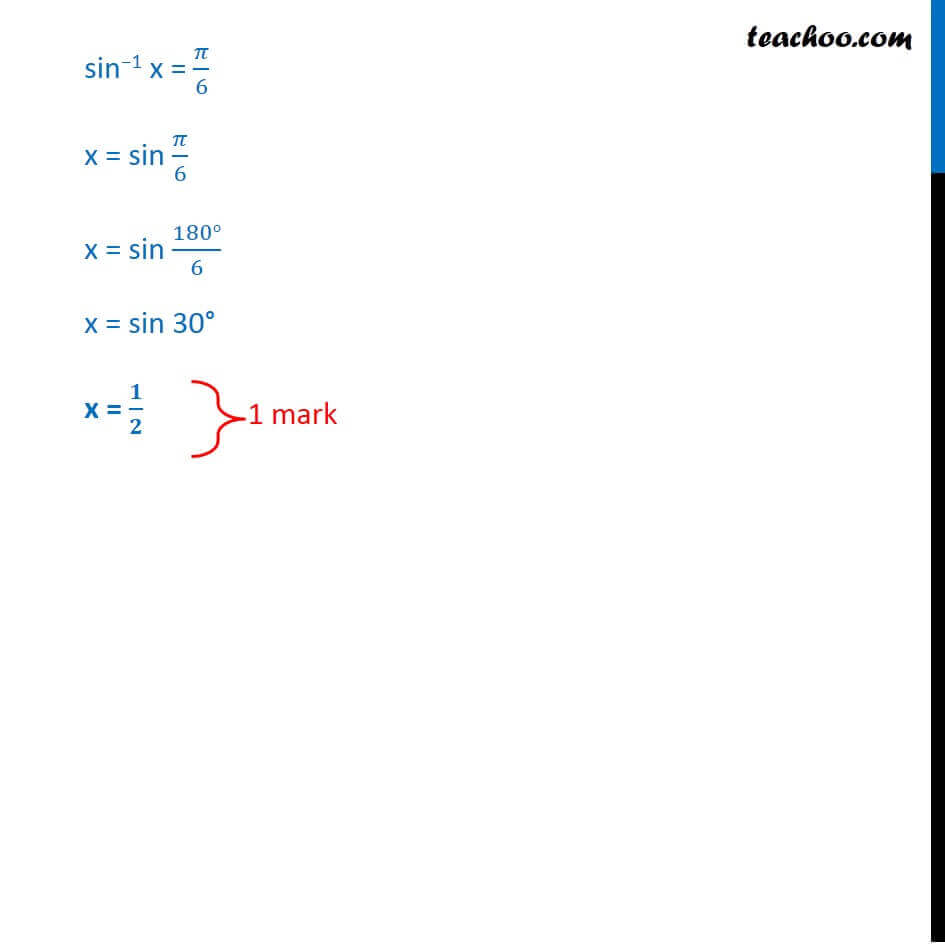CBSE Class 12 Sample Paper for 2018 Boards

Class 12
Solutions of Sample Papers and Past Year Papers - for Class 12 Boards

### If 4sin −1 x + cos −1 x = 𝜋 then find the value of x.

This is a question of CBSE Sample Paper - Class 12 - 2017/18.Learn in your speed, with individual attention - Teachoo Maths 1-on-1 Class

### Transcript

Question 5 If 4sin 1 x + cos 1 x = then find the value of x. 4sin 1 x + cos 1 x = We know that sin 1 x + cos 1 x = /2 cos 1 x = /2 "sin 1 x" 4sin 1 x + / "sin 1 x" = 4sin 1 x "sin 1 x" = /2 3sin 1 x = /2 sin 1 x = 1/3 /2 sin 1 x = /6 sin 1 x = /6 x = sin /6 x = sin (180 )/6 x = sin 30 x = /